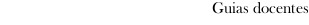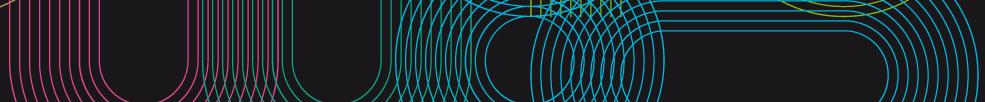Educational guide 2017_18Escola de Enxeñaría Industrial
 Máster Universitario en Enxeñaría IndustrialSubjectsPhysics ExtendedContents
 Topic Sub-topic I.1. WAVE MOTION 1.1. Wave phenomena 1.2. Fundamental characteristics of waves 1.3. The wave equation 1.4. Plane waves 1.5. Wavefront and wavevector 1.6. Cylindrical and spherical waves 1.7. Longitudinal and transverse waves 1.8. Huygens principle 1.9. Reflection and refraction of waves I.2. MECHANICAL WAVES 2.1. The nature of mechanical waves 2.2. Longitudinal waves in thin rods 2.3. Longitudinal waves in springs 2.4. Transverse waves in strings 2.5. Power flow and intensity of a wave 2.6. Longitudinal waves in fluids I.3. DESCRIPTION OF PHYSICAL QUANTITIES BY MEANS OF VECTOR ANALYSIS 3.1. Differential of arc of a curve 3.2. Scalar fields 3.3. Directional derivative 3.4. Gradient 3.5. Vector fields 3.6. Flux of a vector field 3.7. Solenoidal fields 3.8. Divergence of a vector field 3.9. Ostrogradski-Gauss theorem or divergence theorem 3.10. Divergence of a solenoidal field 3.11. Circulation of a vector field 3.12. Rotation or curl of a vector field 3.13. Stokes theorem 3.14. Conservative fields II.1. GENERAL EQUATIONS OF ELECTROMAGNETISM 1.1. Definition of electric and magnetic fields 1.2. Field sources: macroscopic electric charges and currents 1.3. Relations among fields E and B and their sources: Maxwells equations 1.4. Free charge 1.5. Polarization charge 1.6. Electric current 1.7. Polarization current 1.8. Magnetization current 1.9. Maxwells equations in function of fields E, D, B, and H 1.10. Boundary conditions for electromagnetic fields 1.11. Electrodynamic potentials 1.12. The energy law of the electromagnetic field II.2. TIME-INDEPENDENT FIELDS: ELECTROSTATICS, STEADY ELECTRIC CURRENT AND MAGNETOSTATICS 2.1. Fundamental equations of electrostatics 2.2. Electric dipole 2.3. Fundamental equations for steady electric current 2.4. Equations including media properties 2.5. Electrical resistance 2.6. Joules law 2.7. Electromotive forces and generators 2.8. Potential distribution in a resistor 2.9. Fundamental equations of magnetostatics 2.10. Equations including media properties 2.11. Magnetic forces 2.12. Magnetic circuit 2.13. Magnetic dipole II.3. ELECTROMAGNETIC INDUCTION AND QUASISTATIC FIELDS 3.1. Electromagnetism in moving media 3.2. Galilean transformation of electric and magnetic fields 3.3. Electromotive force around a circuit 3.4. Faradays law of electromagnetic induction 3.5. Definition of quasistatic fields 3.6. Self-inductance and mutual inductance 3.7. Magnetic energy II.4. ELECTROMAGNETIC WAVES 4.1. Wave equations for fields E and H 4.2. E.M. monochromatic plane waves in lossless media 4.3. E.M. monochromatic plane waves in lossy media 4.4. Incidence of a plane wave on an interface between two perfect dielectrics 4.5. Incidence of a plane wave on an interface between a perfect dielectric and a conductor III.1 LABS: STRUCTURED ACTIVITY SESSIONS 1.1 Structured activity sessions: - Experimental data processing (approximate quantities, measurement of physical magnitudes, error estimation) - Adequate operation with basic measurement instruments (flex-meter, micrometer, multimeter (analog and digital), oscilloscope) - Laboratory experiments with mechanical or electromagnetic waves (emission and reception of ultrasonic waves, microwaves or light waves, standing waves along one direction, Michelson interferometer) III.2 LABS: UNSTRUCTURED ACTIVITY (OPEN LAB) SESSIONS 2.1. Unstructured activity (open lab) sessions: - A practical problem, formulated with basic initial data, will be assigned to each working team. Then, under the teachers supervision, each team must analyze the problem, select a possible solution and carry it out at the lab - For the open lab problems, diversity of topics and experimental techniques are considered within the field of wave and electromagnetic phenomena, in particular, electric current conduction and electromagnetic induction in quasistatic regime - As a reference, some open lab problems that can be proposed are: measuring the electric field on a weakly conducting sheet, numerical solution of the Laplace equation, measuring the self-inductance of a coil or a solenoid, measuring the mutual inductance of two coils or two solenoids - As an option, the open lab sessions may be replaced by a well-documented piece of work reporting some topic/technique/process/device related to science or technology where wave or electromagnetic phenomena play an essential role. The report must include a model of the problem, clearly identifying the relevant quantities and physical laws
 Universidade de Vigo            | Reitoría | Campus Universitario | C.P. 36.310 Vigo (Pontevedra) | Spain | Tlf: +34 986 812 000